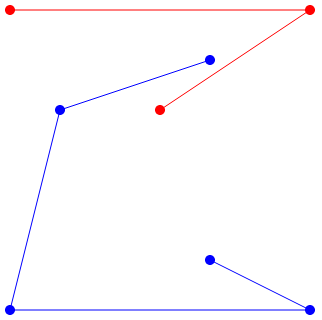CSES - HIIT Open 2019 - Keeping Distance
• Time limit: 1.00 s
• Memory limit: 512 MB
You are given a set of $n$ distinct points in the plane. Each point has integer coordinates between $0 \dots k$, and is either blue or red.

There are always four points with fixed coordinates: $(0,0)$ and $(k,0)$, which are blue, and $(0,k)$ and $(k,k)$, which are red. The other points may have any coordinates and colors, but there are never three points on the same line.

Your task is to add $n-2$ line segments, each of which connects two points of the same color, in such a way that all blue and red points belong to the same component, and no two line segments intersect.

Input

The first input line has two integers $n$ and $k$: the number of points and the coordinate upper bound.

After this, there are $n$ lines that describe the points. Each line has three integers $x$, $y$ and $c$: a point is located at $(x,y)$ and its color is blue ($c=1$) or red ($c=2$).

Output

Print $n-2$ lines that describe the connections. Each line must have four integers $x_1$, $y_1$, $x_2$ and $y_2$: the points $(x_1,y_1)$ and $(x_2,y_2)$ are connected.

You can print any valid solution, and it can be proven that there is always a solution.

Constraints
• $4 \le n \le 1000$
• $1 \le k \le 10^9$
Example

Input:
8 6 0 0 1 0 6 2 1 4 1 3 4 2 4 1 1 4 5 1 6 0 1 6 6 2

Output:
0 0 1 4 0 0 6 0 0 6 6 6 1 4 4 5 6 0 4 1 6 6 3 4

The following figure corresponds to this example: